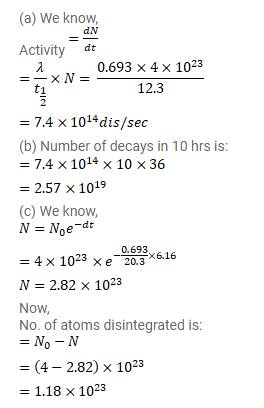# 4×1023 tritium atoms are contained in a vessel.

Question:

$4 \times 10^{23}$ tritium atoms are contained in a vessel. The half-life of decay of tritium nuclei is $12.3 y$. Find (a) the activity of the sample, (b) The number of decays in the next 10 hours (c) the number of decays in the next $6.15 \mathrm{y}$.

Solution: